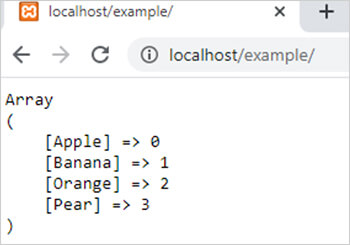array_flip() function in php || PHP Functions

# array_flip() function in php

0 1179

array_flip() function of PHP is used to flip or interchange the values of an array with respective keys means it converts the values into keys and keys into values. It is an inbuilt function of PHP.

Syntax

array_flip (\$arr);

Here,

\$arr is a PHP array.

Example 1:

```<?php
\$arr1= array("fruit1"=>"Apple","fruit2"=>"Banana","fruit3"=>"Orange","fruit4"=>"Pear");
\$result=array_flip(\$arr1);
echo "<pre>";
print_r(\$result);
?>```

Output:Example 2:

array_flip() with indexed array.

```<?php
\$arr1= array("Apple","Banana","Orange","Pear");
\$result=array_flip(\$arr1);
echo "<pre>";
print_r(\$result);
?>```

Output:Share: